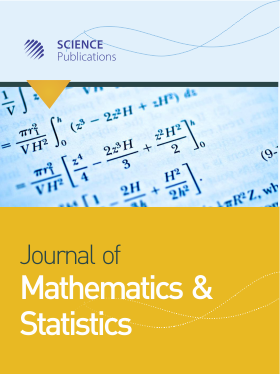Frequency: Continuous
ISSN: 1549-3644 (Print)
ISSN: 1558-6359 (Online)
Research Article Open Access

# Mathematical Approach to the Ruin Problem with Compounding Assets

Mercy Amaebi Orukari1
• 1 Department of Mathematics, Niger Delta University, Nigeria

## Abstract

This study considered the Ruin problem with an income process with stationary independent increments. The characterization is obtained which is general for the probability of $r(y)$, that the asset of a firm will never be zero whenever the initial asset level of the firm is $y$. The aim of this study is also to determine $r(y) = P \{ T < \infty | Y(0) = y \}$, If we let $T = inf \{ t \ge 0; Y(t) < 0 \}$, A condition that is necessary and sufficient is studied for a distribution that is one – dimensional of $X_N$ which coverages to $X_*$. The result that is obtained concerning the probability, is of ruin before time $t$. Riemann-Stieltjes integral, two functions $f$ and with symbol as $\int_a^b f(x)da(x)$ was used and is a special case in which $a() = x$, where $a$ has a continuous derivative. It is defined such that the Stieltjes integral $\int_a^b f(x)da(x)$ becomes the Riemann integral $\int_a^b f(x)a^| d(x)$.

Journal of Mathematics and Statistics
Volume 18 No. 1, 2022, 143-147

DOI:

Submitted On: 26 April 2022 Published On: 8 October 2022

How to Cite: Orukari, M. A. (2022). Mathematical Approach to the Ruin Problem with Compounding Assets. Journal of Mathematics and Statistics, 18(1), 143-147. https://doi.org/10.3844/jmssp.2022.143.147

• 664 Views